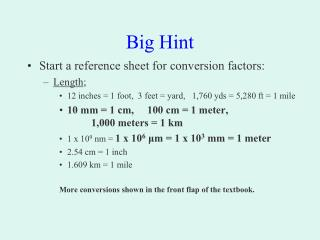DownloadDownload PresentationBig Hint

Big Hint

Télécharger la présentationBig Hint

- - - - - - - - - - - - - - - - - - - - - - - - - - - E N D - - - - - - - - - - - - - - - - - - - - - - - - - - -
Presentation Transcript

1. Big Hint • Start a reference sheet for conversion factors: • Length; • 12 inches = 1 foot, 3 feet = yard, 1,760 yds = 5,280 ft = 1 mile • 10 mm = 1 cm, 100 cm = 1 meter, 1,000 meters = 1 km • 1 x 109 nm = 1 x 106μm = 1 x 103 mm = 1 meter • 2.54 cm = 1 inch • 1.609 km = 1 mile More conversions shown in the front flap of the textbook.

2. Big Hint • Start a reference sheet for conversion factors: • Mass; • 1,000 mg = 1 gram, 1,000 g = 1 kg • 453.6 g = 1 lb More conversions shown in the front flap of the textbook.

3. Big Hint • Start a reference sheet for conversion factors: • Time; • 60 seconds = 1 min, 60 min = 1 hr, 24 hrs = 1 day, 365 days = 1 yr • 3,600 sec = 1 hr More conversions shown in the front flap of the textbook.

4. Big Hint • Start a reference sheet for conversion factors: • Volume; • 1 ml = 1 cm3 • 1 dm3 = 1,000 cm3 = 1 Liter • 1,000 mL = 1 L • 3.786 L = 1 gallon More conversions shown in the front flap of the textbook.

5. Big Hint • Start a reference sheet for conversion factors: • Temperature; • 0°C = 32°F, 100°C = 212°F • °C = (°F - 32) / 1.8 = 5 x (°F - 32) / 9 • K = °C + 273 More conversions shown in the front flap of the textbook.

6. Practice • Convert the following: • 60 mph = ? ft/s

7. Practice • Convert the following: • 77°F = ? K (note, no ° symbol used with K)

8. Practice • Convert the following: • 30 km/h = ? m/s

9. Practice • Convert the following: • 500 mL = ? L

10. Conversions • Conversion Example - 1: • The speedometer on your fancy European car reads 25 m/s, and you are driving in a posted 75 km/hr speed zone. Are you speeding?

11. Conversions • Conversion Example - 2: • A truck driver put 500 liters of diesel fuel (ρ = 0.76 g/cm3) into the fuel tank of his vehicle. In kilograms, how much did the mass of the truck increase?

12. Conversions • Conversion Example - 3: • Change the density of 1 g/cm3 to kg/m3.